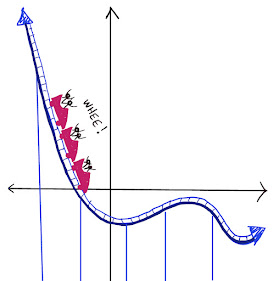## Tuesday, October 20, 2015

### Coincidence? Why are Primes Clustered Around Multiples of 6?

For the solution, click "Read More" below.

Solution:

#### Why are the prime numbers clustered around multiples of 6?Let's consider what happens when we divide an integer by 6.  There are 6 possible remainders, including the remainder of 0, which we get when the number is a multiple of 6:Remainder when we divide by 6  Can the number be prime?0Must be a multiple of 2 and 3.1May be prime, such as 13.  But not always, such as 25.2Must be a multiple of 2.3Must be a multiple of 3.4Must be a multiple of 2.5May be prime, such as 29.  But not always, such as 35.Looking at the chart, we can see that numbers leaving a remainder of 0, 2, or 4 must be a multiple of 2, and numbers leaving a remainder of 0 or 3 must be a multiple of 3.  Other than 2 and 3 themselves, these numbers are all composite.  Therefore all primes greater than 3 must have a remainder of 1 or 5 when we divide by 6.  In other words, they must be one more or one less than a multiple of 6.